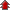Home Board Paper Solutions Text Book Solutions Articles NCERT Exemplar Solutions

# Sets### Exercise 1

•  Q2 Let A = {1, 2, 3, 4, 5, 6}. Insert the appropriate symbol ∈or ∉ in the blank spaces: (i) 5__A (ii) 8__A (iii) 0__A (iv) 4__A (v) 2__A (vi) 10__A Q3 Write the following sets in roster form: (i) A = {x: x is an integer and - 3 < x < 7}. (ii) B = {x: x is a natural number less than 6}. (iii) C = {x: x is a two-digit natural number such that the sum of its digits is 8} (iv) D = {x: x is a prime number which is divisor of 60}. (v) E = The set of all letters in the word TRIGONOMETRY. (vi) F = The set of all letters in the word BETTER. Q4 Write the following sets in the set-builder form: (i) (3, 6, 9, 12) (ii) {2, 4, 8, 16, 32} (iii) {5, 25, 125, 625} (iv) {2, 4, 6 upto infinity} (v) {1, 4, 9, upto 100}

## Recently Viewed Questions of Class 11th mathematics

• NCERT Chapter